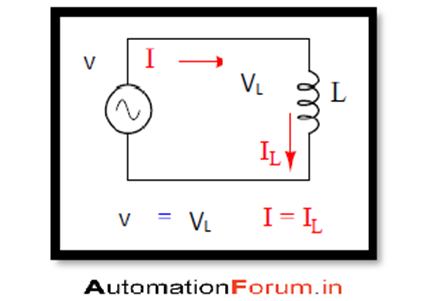# AC THROUGH INDUCTANCE RESISTANCE AND CAPACITANCE

AC THROUGH INDUCTANCE RESISTANCE AND CAPACITANCE

A circuit consists of many different components connected together to form a complete circuit there are three passive components which is mostly used in circuits they are capacitor-inductor and resistor and these components have one common thing they will slow down the flow of electric current in a circuit and each of them does this process in a different way. The flow of electric current is done in two ways the Dc current will flow in one steady direction while the AC current alternates in both directions

INDUCTIVE CIRCUITThe inductor function is different from resistors, the resistors normally opposes the current through them while in case of the inductors it opposes the changes in current through them, by dropping the voltage it will react to the change in current and thus it opposes the current change and the voltage dropping will be directly proportional to the rate of change of the current so that it can maintain the current at its present value, if the magnitude of the current increases it will oppose it and if the current is decreasing it will reverse the polarity and opposes the decrease this opposition of the current change is called as reactance

CAPACITIVE CIRCUIT

In case of capacitive circuit if an alternating voltage is applied to the capacitor it first charges in one direction and then in the opposite, capacitor opposes the changes in voltage in a circuit and it is done by drawing or supplying the current as it will charge or discharge to the new voltage level. The rate of change of voltage across the capacitor is directly proportional to the flow of electrons

The current through the capacitor is a reaction against the change in voltage across it so if the instantaneous voltage is at peak the current will be zero and the current will be maximum if the instantaneous voltage is at maximum change

RESISTIVE CIRCUIT

In a circuit the resistor will oppose the flow of electrons through them, the resistor will impose a voltage reduction as a result of the current flow. If the voltage is negative the power will be positive and by the polarity of the power we can say that resistor is always dissipating power, and the power will be taken from the source and it will be released in the form of heat energy